Difference between revisions of "Comparative advantage"

Definition

The comparative advantage of an organization (individual, firm, or country) is in the activity that the organization can do with the maximum difference between the benefit and the opportunity cost. In other words, it is what the individual or country has the most advantage relative to other things the individual or country can do, and relative to things that others can do.

If organizations choose to work according to their comparative advantage, then social utility (and hence, social surplus) is maximized. In principle, comparative advantage can emerge both in a market economy (through the effect of gains from trade) and in a centrally planned economy.

Note that the concept of comparative advantage best makes sense for organization that already have an independent reason to exist, and cannot be used to justify new organizations into existence.

Example

A simple example involving two persons and two tasks

Suppose an economy has two individuals$A$ and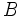$B$ and two activities$M$ and$N$. Further, suppose that:

•$A$ can generate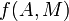$f(A,M)$ units of worth per hour doing$M$ and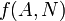$f(A,N)$ units per hour doing$N$.
•$B$ can generate$f(B,M)$ units of worth per hour doing$M$ and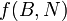$f(B,N)$ units per hour doing$N$.

Suppose, further, that each person can choose to do exactly one of the two tasks, and they must do different tasks.

Since each can choose to do exactly one task, and they must do different tasks, there are two possible assignments:

•$A$ does$M$ and$B$ does$N$, yielding a total of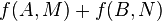$f(A,M) + f(B,N)$.
•$A$ does$N$ and$B$ does$M$, yielding a total of$f(A,N) + f(B,M)$.

If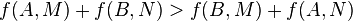$f(A,M) + f(B,N) > f(B,M) + f(A,N)$, then the first allocation is optimal. If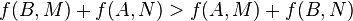$f(B,M) + f(A,N) > f(A,M) + f(B,N)$, then the second allocation is optimal. This has three equivalent interpretations:

Condition for$A$ to do$M$ and$B$ to do$N$ Verbal formulation of interpretation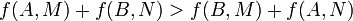$\!f(A,M) + f(B,N) > f(B,M) + f(A,N)$ We are comparing the total output generated under the two possible configurations, and choosing the one that generates greater output.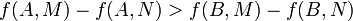$\!f(A,M) - f(A,N) > f(B,M) - f(B,N)$ Here, we are treating$N$ as the alternative activity and comparing (Between$A$ and$B$, the gain in doing$M$ over and above the opportunity cost.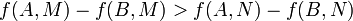$\! f(A,M) - f(B,M) > f(A,N) - f(B,N)$ Here, we are comparing the competitive advantage of$A$ over$B$ in both$M$ and$N$ and allocating to$A$ the activity where the competitive advantage is greater.

Comparative advantage differs from two other possible allocation rules: each person does what that person is best at and each activity is done by the person who is best at that activity. The problem with the first allocation rule is that it may allocate both people to the same task, and the problem with the second allocation rule is that it may allocate both tasks to a single person. On the other hand, the law of comparative advantage always makes sense and maximizes total output.

Note, however, that if either of those rules does give a unique allocation, then that agrees with the allocation given by comparative advantage.

These two rules are contrasted with comparative advantage below:

Informal description of rule Mathematical condition for$A$ to do$M$,$B$ to do$N$ Corresponding description of comparative advantage rule Key difference
Each person does what that person is best at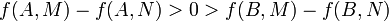$\! f(A,M) - f(A,N) > 0 > f(B,M) - f(B,N)$$\! f(A,M) - f(A,N) > f(B,M) - f(B,N)$ Comparative advantage is only a comparative rule and does not require anything about the signs of the expressions$f(A,M) - f(A,N)$ and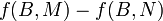$f(B,M) - f(B,N)$
Each activity is done by the person best at that activity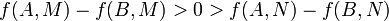$\! f(A,M) - f(B,M) > 0 > f(A,N) - f(B,N)$$\! f(A,M) - f(B,M) > f(A,N) - f(B,N)$ Comparative advantage is only a comparative rule and does not require anything about the signs of the expressions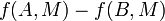$f(A,M) - f(B,M)$ and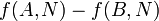$f(A,N) - f(B,N)$

Here are some examples to illustrate how different rules would give different predictions:

Mathematical condition Numerical values that illustrate it Comparative advantage says ... Each individual doing what that individual is best at gives ... Each activity done by the person best at it gives ...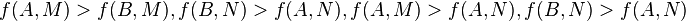$\! f(A,M) > f(B,M), f(B,N) > f(A,N), f(A,M) > f(A,N), f(B,N) > f(A,N)$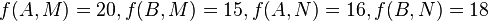$\! f(A,M) = 20, f(B,M) = 15, f(A,N) = 16, f(B,N) = 18$$A$ does$M$,$B$ does$N$$A$ does$M$,$B$ does$N$$A$ does$M$,$B$ does$N$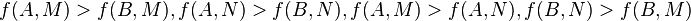$\! f(A,M) > f(B,M), f(A,N) >f(B,N), f(A,M) > f(A,N), f(B,N) > f(B,M)$$\! f(A,M) = 20, f(B,M) = 15, f(A,N) = 18, f(B,N) = 16$$A$ does$M$,$B$ does$N$$A$ does$M$,$B$ does$N$ This rule does not work since it would allocate$A$ with both$M$ and$N$.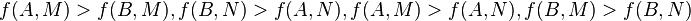$\! f(A,M) >f(B,M), f(B,N) > f(A,N), f(A,M) > f(A,N), f(B,M) > f(B,N)$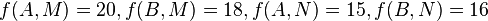$\! f(A,M) = 20, f(B,M) = 18, f(A,N) = 15, f(B,N) = 16$$A$ does$M$,$B$ does$N$ This rule does not work since both$A$ and$B$ would choose$M$$A$ does$M$,$B$ does$N$

A more sophisticated example involving marginal costs and marginal utilities

In the previous example, we assumed that each person could choose to do exactly one task, and the two persons had to choose different tasks. If this constraint were absent, each person would simply do what he or she were best at, and the comparative advantage phenomenon may not arise.

Although a rigid limit on the number of people who can do each task may not exist in real life, there are a number of realistic features that could give rise to the same observations. These include:

• Increasing marginal cost of production (typically, in terms of some other factor of production) for each type of activity, that makes it worthwhile, after a certain point, to start doing another activity. For instance, there may be some land that is suitable for growing wheat and some land that is suitable for growing rice. Using land suitable for one crop to grow another is counterproductive. This leads to a tendency for some farmers to grow wheat and others to grow rice. Who chooses to grow which crop is then determined by comparative advantage. A similar case can be made when the capital stock for each type of productive activity is limited.
• Decreasing marginal utility: A society may gain a lot of utility from a bit of this and a bit of that, but less utility if only one type of good is produced. This has to do with the fact that the marginal utility from having more of a good is decreasing: the more there is, the less additional quantity of the good yields worth.

Implementation of comparative advantage

Comparative advantage in a market economy

In a market economy, where the goods produced are easy to trade, people tend to work in areas where they have comparative advantage, rather than what they are best at. This is because the loss they incur by not doing something they are more productive at is more than compensated by the gain they get from trading with somebody who is even better at that task.

Comparative advantage, division of labor, and specialization

The basic definition and formulation of comparative advantage does not depend on any assumption about greater gain in skills due to specialization. Comparative advantage suggests a division of labor even without the benefits of skill gains that may arise from specialization.